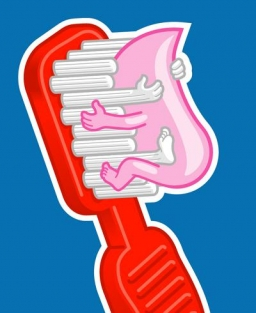# Dentist

Class 5. I participated in a preventive dental examination. A quarter of the pupils found two dental caries, and an eighth found one caries. Half the class had all their teeth healthy. How many students attend class 5? If four students were missing on the day of the dental examination?

n =  32

### Step-by-step explanation:

n/4+n/8 + n/2 + 4 = n

n = 32

n = 32/1 = 32

n = 32

Our simple equation calculator calculates it.Did you find an error or inaccuracy? Feel free to write us. Thank you!

Tips for related online calculators
Do you have a linear equation or system of equations and looking for its solution? Or do you have a quadratic equation?